Courses
Courses for Kids
Free study material
Free LIVE classes
More

# 4 - Digit Addition and Subtraction with Borrowing for Class 4LIVE
Join Vedantu’s FREE Mastercalss

4-digit addition refers to the addition of numbers in which either one or both the addends are of 4 digits. The procedure of 4-digit addition is the same as 3-digit addition, with just an increase in the number of digits in the addends. 4-digit subtraction refers to the subtraction of numbers in which the minuend is of 4 digits, and the subtrahend is either of 1, 2, or 3 digits. In 4-digit subtraction, we subtract the numbers one by one according to their place values: ones, tens, hundreds, and thousands.

Addition with regrouping or addition with carryover refers to additions where the addends digit sums exceed 9 in any of the columns. Finding the digit sum of two integers requires regrouping. Only the units place digit of the total is then written in the appropriate column. After that, we immediately move the tens place digit to the column to the left and carry on with the addition. Let's use an example to clarify 4-digit addition with regrouping.

Add the 4-digit numbers: 3195 and 6237.

Ans: Step 1: Add the numbers in the ones column. 5 + 7 = 12. Write 2 in ones column and carry 1 to the tens column. (Regrouping)

Step 2: Add the numbers in the tens column. 9 + 3 + 1 (carryover) = 13. Write 3 in tens column and carry 1 to the hundreds column. (Regrouping)

Step 3: Add the numbers in the hundreds column. 1 + 2 + 1 (carryover) = 4. Write 4 in the hundreds column.

Step 4: Add the numbers in the thousands column. 3 + 6 = 9 thousands. Write 9 in the thousands column.

Thus, the addition of 4-digit numbers 3195 and 6237 results in the sum 9432.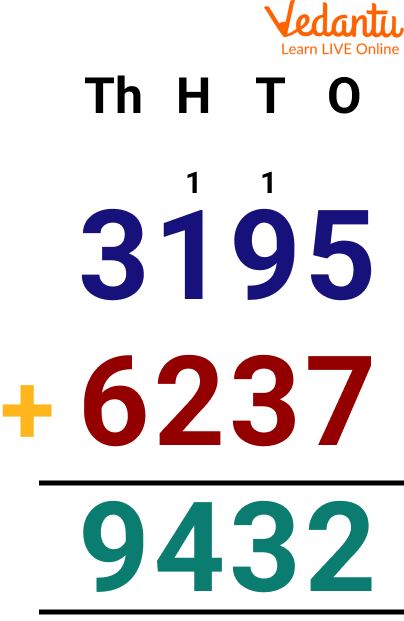While carrying out 4-digit addition, if the sum of the digits of the addends is less than or equal to 9, this addition is called 4-digit addition without regrouping or without carrying. Let us understand 4-digit addition with the help of an example.

Use the following steps to add the 4-digit numbers: 6134 and 2423.

Step 1: Add the numbers under the ones column. 4 + 3 = 7. Write 7 in ones column.

Step 2: Add the numbers under the tens column. 3 + 2 = 5. Write 5 in the tens column.

Step 3: Add the numbers under the hundreds column. 1 + 4 = 5. Write 5 in the hundreds column.

Step 4: Add the numbers under the thousands column. 6 + 2 = 8. Write 8 in the thousands column.

It should be noted that the sum of each column is less than 9 so there is no regrouping. Thus, the addition of the 4-digit numbers 6134 and 2423 results in the sum 8557.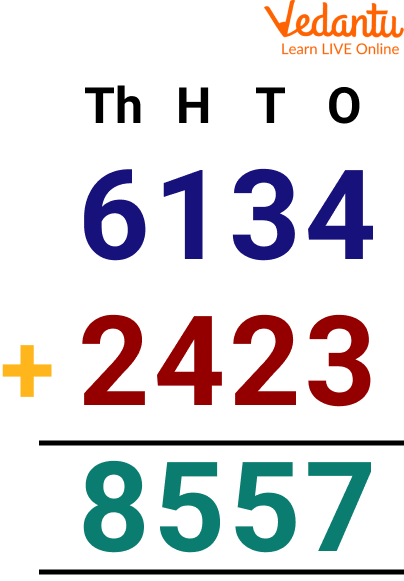## Four-digit Subtraction with Borrowing

4-digit subtraction with borrowing is also known as 4-digit subtraction with regrouping. When any digit in the minuend (top row) is smaller than the corresponding digit in the subtrahend (bottom row), we regroup the numbers and then subtract. While regrouping, we borrow 1 number from the preceding column to make that particular digit in the minuend bigger than the corresponding digit of the subtrahend. Let us understand this with an example.

Example: Subtract 3678 from 8162.

Step 1: Arrange the numbers according to their place value. Start subtracting the digits in ones column. We can see that 2 is smaller than 8. So, we will borrow 1 from the tens column, making it 12. Now, 12 - 8 = 4.

Step 2: After giving 1 to the ones column in the previous step, 6 becomes 5. Now, let us subtract the digits in the tens place (5 - 7). Here, 5 is smaller than 7. So, we will borrow 1 from the hundreds column. This will make it 15. So, 15 - 7 = 8.

Step 3: In step 2 we had given 1 to the tens column, so we have 0 in the hundreds place. Here, 0 is smaller than 6, so we will borrow 1 from the thousands column. This will make it 10. So, 10 - 6 = 4.

Step 4: Now, let us subtract the digits in the thousands place. After giving 1 to the hundreds column, 8 becomes 7. So, 7 - 3 = 4.

Therefore, the difference between the two given numbers is, 8162 - 3678 = 4484.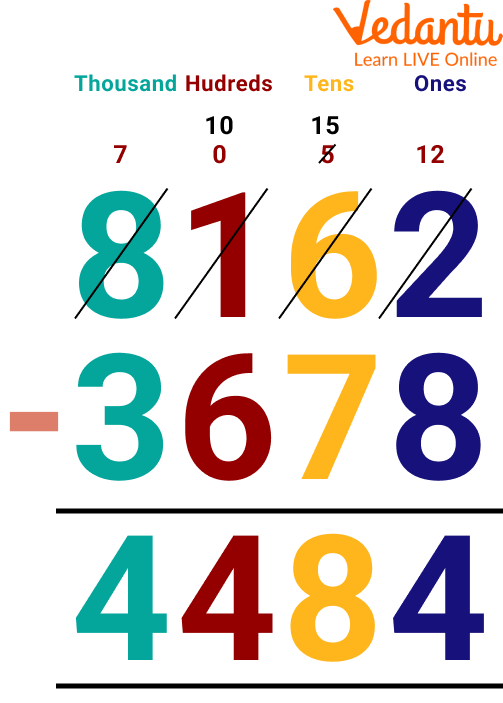Four-digit Subtraction with Borrowing

## Solved Examples

Q 1: Subtract the 4-digit numbers: 4572 - 3127.

Ans: Let us subtract 3127 from 4572.

Step 1: Arrange the numbers according to their place value.

Step 2: We start subtracting from the ones column. Since 2 is smaller than 7, we will borrow 1 from the tens column, making it 12. Now, 12 - 7 = 5.

Step 3: After giving 1 to the ones column in the previous step, 7 becomes 6. Now, subtract the digits in tens place. So, 6 - 2 = 4

Step 4: Moving on, we subtract the digits in the hundreds place. So, 5 - 1 = 4

Step 5: Subtract the digits in the thousands place. So, 4 - 3 = 1

Therefore, the difference between the given numbers is 1445.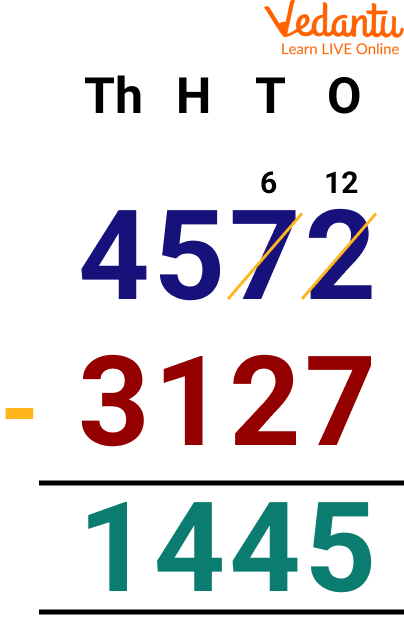Subtract the 4-digit numbers: 4572 - 3127.

Q 2. Add the given 4-digit numbers: 4472 and 5227.

Ans: Let us do the 4-digit addition of the given numbers using the following steps.

Step 1: Add the numbers in the ones column. 2 + 7 = 9. Write 9 in ones column.

Step 2: Add the numbers in the tens column. 7 + 2 = 9. Write 9 in tens column.

Step 3: Add the numbers in the hundreds column. 4 + 2 = 6. Write 6 in the hundreds column.

Step 4: Add the numbers in the thousands column. 4 + 5 = 9. Write 9 in the thousands column.

Thus, the addition of the 4-digit numbers 4472 and 5227 results in the sum 9699.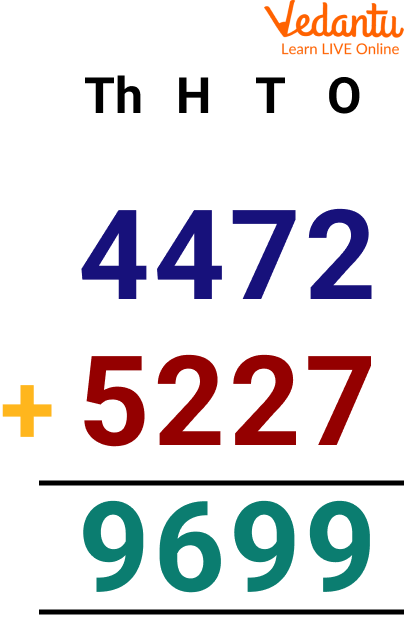Add the given 4-digit numbers: 4472 and 5227.

## Practice Problems

Q1 Subtract 4617 from 8878.

Ans. 4261

Q2 Subtract 2615 from 6745.

Ans. 3860

Ans. 5953

## Summary

To conclude this article on subtraction with borrowing for class 4, we will say that subtraction is an arithmetic operation if we remove the smaller number from the larger number. In some cases, as we have seen in the article, we do four-digit subtraction with borrowing.

We have learnt the borrowing method in this article. We also learnt how to subtract one number from the other. We also practised subtraction with solved examples and practice problems. We hope you enjoyed reading this article.

Last updated date: 28th Sep 2023
Total views: 78.6k
Views today: 2.78k

## FAQs on 4 - Digit Addition and Subtraction with Borrowing for Class 4

1. What is another word that can be used in place of subtraction?

Difference or takeaway can be used in place of subtraction.

2. What are the 4th-grade subtraction strategies?

Once students understand that if they know the addition fact 9+9=18, then they also know the subtraction fact 18-9=9, they can begin to apply this strategy to 'near doubles' subtraction problems such as 11-5, 42-20 or 298-150. With this strategy, they can solve the problem easily.

3. Will the commutative property apply to subtraction?

Commutative property is the property where we change the sequence of the numbers to be added, but the sum remains the same. This property can't be applied to subtraction. This property can also be used in 4-digit addition with regrouping and 4-digit addition without regrouping.